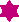# User Forum

Subject :NSO    Class : Class 9

4The speed of a train increases at a constant rate a from zero to v, and then remains constant for an interval, and finally decreases to zero at a constant rate b. If L be the total distance travelled, then the total time taken is

(A)(B)(C)(D)